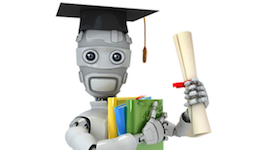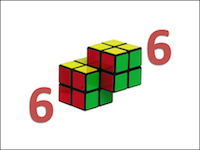# Preparations for DS/AI/ML/Quant

## What is this

A short list of resources and topics covering the essential quantitative tools for data scientists, AI/machine learning practitioners, quant developers/researchers and those who are preparing to interview for these roles.

At a high-level we can divide things into 3 main areas:

1. Machine Learning
2. Coding
3. Math (calculus, linear algebra, probability, etc)

Depending on the type of roles, the emphasis can be quite different. For example, AI/ML interviews might go deeper into the latest deep learning models, while quant interviews might cast a wide net on various kinds of math puzzles. Interviews for research-oriented roles might be lighter on coding problems or at least emphasize on algorithms instead of software designs or tooling.

## List of resources

A minimalist list of the best/most practical ones:Machine Learning:

• Course on classic ML: Andrew Ng’s CS229 (there are several different versions, the Cousera one is easily accessible. I used this older version)
• Book on classic ML: Alpaydin’s Intro to ML link
• Course with a deep learing focus: CS231 from Stanford, lectures available on Youtube.

If you are just breaking into the field I think the above are enough, stop there and move on to other areas of preparation. Here are a few very optional items, mostly on deep learning, in case you have more time:

• Overview book on deep learning: [Deep Leanring] (https://www.deeplearningbook.org/) by Ian Goodfellow et al.
• Amazing book on deep laerning NLP: Yoav Goldberg’s Neural Network Methods for Natural Language Processing
• Pick one of those Udacity nanodegrees on deep learning / self-driving cars
• Hands on exercises on deep learning: Pytorch and MXNet/Gluon are easier to pick up compared to Tensorflow. For anyone of them, you can find plenty of hands on examples online. My biased recommendation is https://d2l.ai/ using MXNet/Gluon created by people at Amazon (it came from mxnet-the-straight-dope)

Coding:

Math:

• Calculus and Linear Algebra: undergrad class would be the best, refresher notes from CS229 link
• Probability: Harvard Stats110 link; book from the same professor.
• Statistics: Shaum’s Outline link.
• [Optional] Numerical Methods and Optimization: these are two different topics really, college courses are probably the best bet. I have yet to find good online courses for them. But don’t worry, most interviews won’t really touch on them.

## List of topics

Here is a list of topics from which interview questions are often derived. The depth and trickiness of the questions certainly depend on the role and the company.

Under topic I try to add a few bullet points of the key things you should know.

### Machine learning

• Models (roughly in decreasing order of frequency)
• Linear regression - e.g. assumptions, multicollinearity, derive from scratch in linear algebra form
• Logistic regression
• be able to write out everything from scratch: from definitng a classficiation problem to the gradient updates
• Decision trees/forest - e.g. how does a tree/forest grow, on a pseudocode level
• Clustering algorithms
• e.g. K-means, agglomerative clustering
• SVM
• e.g. margin-based loss objectives, how do we use support vectors, prime-dual problem
• Generative vs discriminative models
• e.g. Gaussian mixture, Naive Bayes
• Anomaly/outlier detection algorithms (DBSCAN, LOF etc)
• Matrix factorization based models
• Training methods
• Gradient descent, SGD and other popular variants - Understand momentum, how they work, and what are the diffrences between the popular ones (RMSProp, Adgrad, Adadelta, Adam etc) - Bonus point: when to not use momentum?
• EM algorithm - Andrew’s lecture notes are great, also see this
• Learning theory / best practice (see Andrew’s advice slides)
• Bias vs variance, regularization
• Feature selection
• Model validation
• Model metrics
• Ensemble method, boosting, bagging, bootstraping
• Generic topics on deep learning

### Coding essentials

The bare minimum of coding concepts you need to know well.

• Data structures:
• array, dict, link list, tree, heap, graph, ways of representing sparse matrices
• Sorting algorithms:
• see this from brilliant.org
• Tree/Graph related algorithms
• Traversal (BFS, DFS)
• Shortest path (two sided BFS, dijkstra)
• Recursion and dynamic programming

### Calculus

Just to spell things out

• Derivatives
• Product rule, chain rule, power rule, L’Hospital’s rule,
• Partial and total derivative
• Things worth remembering
• common function’s derivatives
• limits and approximations
• Applications of derivatives: e.g. this
• Integration
• Power rule, integration by sub, integration by part
• Change of coordinates
• Taylor expansion
• Single and multiple variables
• Taylor/McLauren series for common functions
• Derive Newton-Raphson
• ODEs, PDEs (common ways to solve them analytically)

### Linear algebra

• Vector and matrix multiplication
• Matrix operations (transpose, determinant, inverse etc)
• Types of matrices (symmetric, Hermition, orthogonal etc) and their properties
• Eigenvalue and eigenvectors
• Matrix calculus (gradients, hessian etc)
• Useful theorems
• Matrix decomposition
• Concrete applications in ML and optimization

### Probability

Solving probability interview questions is really all about pattern recognition. To do well, do plenty of exercise from this and this. This topic is particularly heavy in quant interviews and usually quite light in ML/AI/DS interviews.

• Basic concepts
• Event, outcome, random variable, probability and probability distributions
• Combinatorics
• Permutation
• Combinations
• Inclusion-exclusion
• Conditional probability
• Bayes rule
• Law of total probability
• Probability Distributions
• Expectation and variance equations
• Discrete probability and stories
• Continuous probability: uniform, gaussian, poisson
• Expectations, variance, and covariance
• Linearity of expectation
• solving problems with this theorem and symmetry
• Law of total expectation
• Covariance and correlation
• Independence implies zero correlation
• Hash collision probability
• Universality of Uniform distribution
• Proof
• Circle problem
• Order statistics
• Expectation of min and max and random variable
• Graph-based solutions involving multiple random variables
• e.g. breaking sticks, meeting at the train station, frog jump (simplex)
• Approximation method: Central Limit Theorem
• Approximation method: Poisson Paradigm
• Definition, examples (duplicated draw, near birthday problem)
• Poisson count/time duality
• Poisson from poissons
• Markov chain tricks
• Various games, introduction of martingale

### Statistics

• Z-score, p-value
• t-test, F-test, Chi2 test (know when to use which)
• Sampling methods
• AIC, BIC

### [Optional] Numerical methods and optimization

• Computer errors (e.g. float)
• Root finding (newton method, bisection, secant etc)
• Interpolating
• Numerical integration and difference
• Numerical linear algebra
• Solving linear equations, direct methods (understand complexities here) and iterative methods (e.g. conjugate gradient)
• Matrix decompositions/transformations (e.g. QR, LU, SVD etc)
• Eigenvalue (e.g. power iteration, Arnoldi/Lanczos etc)
• ODE solvers (explicit, implicit)
• Finite-difference method, finite-element method
• Optimization topics: TBA